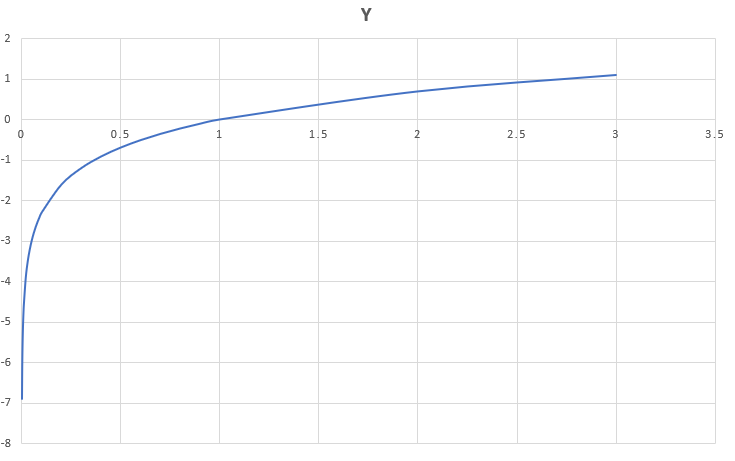Open in App
Not now

# log() function in C++

• Difficulty Level : Basic
• Last Updated : 18 Aug, 2022

Log() function in C++ : The log() function in C++ returns the natural logarithm (base-e logarithm) of the argument passed in the parameter.Syntax for returning natural logarithm: result = log(x)

Syntax for returning logarithm (base-10 logarithm) of the argument. result = log10(x)

The parameters can be of any data type like int, double or float or long double. Log() function returns value according to the following conditions are as follows:

```a) if x>1 then positive ..
b) if 0<x<1 returns a negative value ..
c) if x=1 then it returns 0 ..
d) if x=0 then it returns -inf ..
e) if x<0 then it returns NaN(not a number) ```

## CPP

 `// CPP program to implement log() function` `#include ` `using` `namespace` `std;`   `// function to evaluate natural logarithm base-e` `double` `valueE(``double` `d)` `{` `    ``return` `log``(d);` `}`   `// function to evaluate logarithm base-10` `double` `value10(``double` `d)` `{` `    ``return` `log10``(d);` `}`   `// driver program to test the above function` `int` `main()` `{` `    ``double` `d = 10;` `    ``cout << "The logarithm value(base-e) of " << d ` `         ``<< " is " << valueE(d) << endl;` `    ``cout << "The logarithm value(base-10) of " << d ` `         ``<< " is " << value10(d) << endl;` `    ``return` `0;` `}`

Output:

```The logarithm value(base-e) of 10 is 2.30259
The logarithm value(base-10) of 10 is 1```

Time Complexity: O(1)

Auxiliary Space: O(1)

Application: One of the applications of log() function is to calculate values related to log, for e.g. while finding polite number we need the formula to be written in code, for that we can use log() function. Given below is an implementation of log() function.

## CPP

 `// CPP program to find Nth polite number`   `#include& lt; bits / stdc++.h & gt;`   `using` `namespace` `std;`   `// function to evaluate n-th polite number` `double` `polite(``double` `n)` `{` `    ``n += 1;` `    ``double` `base = 2;` `    ``return` `n` `           ``+ (``log``((n + (``log``(n) / ``log``(base))))) / ``log``(base);` `}`   `// driver code` `int` `main()` `{` `    ``double` `n = 7;` `    ``cout& lt;` `    ``<` `    ``(``int``)polite(n);` `    ``return` `0;` `}`

Output:

`11 `

Time Complexity: O(1)

Auxiliary Space: O(1)

My Personal Notes arrow_drop_up
Related Articles